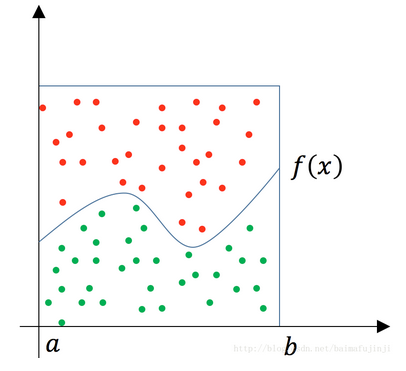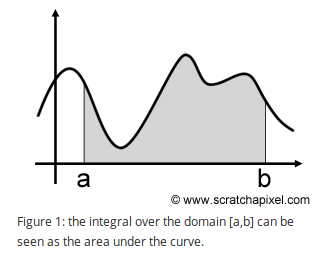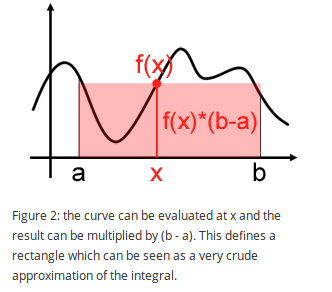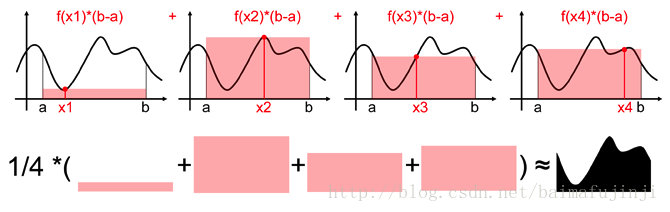# 预备知识

无意识统计学家法则（LOTUS），描述了已知随机变量$X$的概率分布，但不知道$g(X)$的分布，如何求解$g(X)$的数学期望就是LOTUS的目的。 我想有一定概率论基础的同学都应该知道LOTUS的公式：

$X$是离散分布时:

$X$是连续分布时:

其中，$f_X(x)$$x$的概率密度函数（PDF）。总结一下就是在计算期望时，用已知的$X$的PDF代替未知的$g(X)$的PDF。

# 蒙特卡洛求定积分（一）：投点法

这个方法也常常被用来求$\pi$值（我们后续详细描述），现在我们用它来求函数的定积分。如下图所示，有一个函数$f(x)$，若要求它从$a$$b$的定积分， 其实就是求曲线下方的面积。这时我们可以用一个比较容易算得面积的矩型罩在函数的积分区间上（假设其面积为$S_{area}$）。然后随机地向这个矩形框里面投点， 其中落在函数$f(x)$下方的点为绿色，其它点为红色。然后统计绿色点的数量占所有点（红色+绿色）数量的比例即为$\frac{number_{green}}{number_{green} + number_{red}} = r$， 那么就可以据此估算出函数$f(x)$$a$$b$的定积分为$S_{area} \times r$注意由蒙特卡洛法得出的值并不是一个精确之，而是一个近似值。而且当投点的数量越来越大时，这个近似值也越接近真实值。

# 蒙特卡洛求定积分（二）：期望法

接下来我们重点介绍一下利用蒙特卡洛法求定积分的第二种方法——期望法，有时也成为平均值法。

积分的几何意义就是$[a,b]$区间内曲线下方的面积，如下图所示：当我们在$[a,b]$之间随机取一点$x$时，它对应的函数值就是$f(x)$，然后便可以用$f(x)\times (b-a)$来粗略估计曲线下方的面积（也就是积分）， 当然这种估计（或近似）是非常粗略的，过程如下图所示：于是我们想到在$[a,b]$之间随机取一系列点$x_i$$x_i$满足均匀分布），然后把估算出来的面积取平均来作为积分估计的一个更好的近似值。 可以想象，如果这样的采样点越来越多，那么对于这个积分的估计也就越来越接近。按照上面这个思路，我们得到积分公式为:

注意其中的$\displaystyle\frac{1}{b-a}$就是均匀分布的PDF。

任取一组相互独立、同分布的随机变量$\{X_i\}$$Xi$$[a,b]$上服从分布律$f_X$，也就是说$f_X$是随机变量$X$的PDF， 令$g^*(x)=\displaystyle \frac{g(x)}{f_X(x)}$，则$g^*(X_i)$也是一组独立同分布的随机变量，而且因为$g^*(x)$是关于$x$的函数， 所以根据LOTUS可得:

也就是$\bar I$依概率1收敛到$I$。而我们说的平均值法就用$\bar I$作为$I$的近似值。

则定积分$\int_a^b g(x)\mathrm {d}x$就可以用$g^*(x)=\displaystyle \frac{g(x)}{f_X(x)}$期望表示，当$N$越大，其值就越近似于定积分。

如果$f_X$$[a,b]$上服从均匀分布，即：

这和上面的直观解释得到的结果是一样的，再次说明了期望法蒙特卡洛求定积分是可行的。

# 总结

用蒙特卡洛模拟法计算定积分具有普遍意义。蒙特卡洛模拟方法为我们提供了一个看待世界的新思路，即一个不具随机性的事件可以通过一定的方法用随机事件来模拟或逼近。